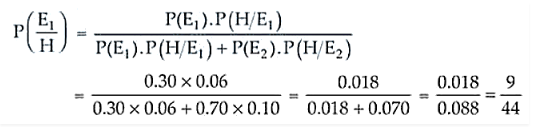# Suppose that 6% of the people with blood group O are left-handed and 10%

Question:

Suppose that 6% of the people with blood group O are left-handed and 10% of those with other blood groups are left-handed 30% of the people have blood group

O. If a left-handed person is selected at random, what is the probability that he/she will have blood group O?

Solution:

Let’s assume

E1 = The event that a person selected is of blood group O

E2 = The event that the people selected is of other group

And H = The event that selected person is left handed

Now,

P(E1) = 0.30 and P(E2) = 0.70

Further, P(H/E1) = 0.06 and P(H/E2) = 0.10

Using Baye’s Theorem, we haveTherefore, the required probability is 9/44.